The center of mass

The center of gravity of an object is the point from which you can suspend an object at rest, and, no matter how the object is oriented, gravity will not cause it to start rotating.  If you suspend an object from any point and let it come to rest, the center of gravity will lie somewhere on a vertical line that passes through the point of suspension.  Near the surface of the earth, where the gravitational acceleration is (nearly) constant, the center of gravity coincides with the center of mass.

Assume a system consist of a collection of particles, for example the atoms that make up a solid object.  The mass of the ith particle is mi and its position coordinates are (xi, yi, zi).  The coordinates of the center of mass (CM) are given below.

xCM = Σmixi/M,  yCM = Σmiyi/M,  zCM = Σmizi/M.

Here M is the total mass of the system.

M = Σmi.

The ∑ symbol stand for sum.  The sum is over all the particles that make up the system.

Newton's 2nd law, F = ma, when applied to an extended object, predicts the motion of a particular reference point for this object.  This reference point is called the center of mass.  The center of mass of a system moves as if the total mass of the system were concentrated at this special point.  It responds to external forces as if the total mass of the system were concentrated at this point.

How do we find the center of mass (CM) of of an object?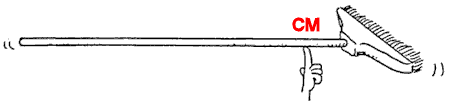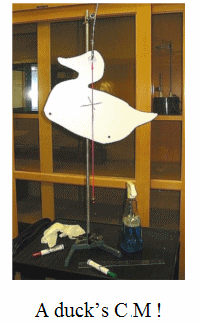Every extended object has a center of mass.  If near the surface of Earth an object, in any orientation, is supported at a location directly below its center of mass or suspended from a location directly above its center of mass, it will be balanced and it will not start to rotate.

Usually, but not always, the center of mass of an object lies within the object itself.  For example, the center of mass of a ball is the very middle of the ball, and the center of mass of a book is the middle of the book.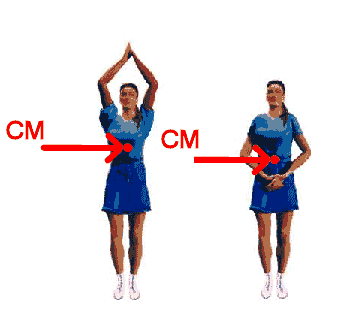If an object has parts that can move with respect to each other, the location of the center of mass depends on the positions of the parts.  For example, when a cheerleader lifts her arms, her center of mass moves to a higher position in her body than when her arms are at her side.

Where is the center of mass (CM) of a person?

In most men, the center of mass is located at or slightly above the navel, in most women it is located below the navel, closer to the hips.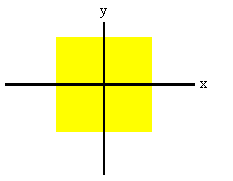For a homogeneous, symmetrical system, the center of mass always lies on the symmetry axis.
Consider a square with sides of length a.  We can position our coordinate system as shown.
The x and y axes are symmetry axes.  We can easily verify that xCM = 0, yCM = 0 from symmetry.
The center of mass lies on the intersection of the symmetry axes, at the origin.

Problem:

Four objects are situated along the y-axis as follows: a 2 kg object is at +3 m, a 3 kg object is at +2.5 m, a 2.5 kg object is at the origin, and a 4 kg object is at -0.5 m.  Where is the center of mass of these objects?

Solution:

• Reasoning:
The coordinates of the center of mass (CM) of a system of objects are
xCM = Σmixi/M,  yCM = Σmiyi/M,  zCM = Σmizi/M.
Here M is the total mass of the system,  M = Σmi.
• Details of the calculation:
The total mass of the system is M = (2 + 3 + 2.5 + 4) kg = 11.5 kg.
yCM = (2 kg * 3 m + 3 kg * 2.5 m + 2.5 kg * 0 m - 4 kg * 0.5 m)/(11.5 kg) = 1 m.
xCM = zCM = 0.

Problem: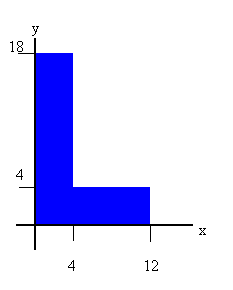A uniform carpenter's square has the shape of an L, as shown in the figure.  Locate the center of mass relative to the origin of the coordinate system.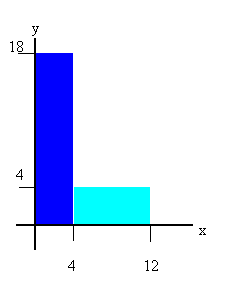Solution:

• Reasoning:
We can think of the system as being made up of two subsystems, as shown in the figure.
The CM of the left subsystem lies at xCM = 2 cm, yCM = 9 cm.  The CM of the right subsystem lies at xCM = 8 cm, yCM = 2 cm.  If the mass of a 1 cm by 1 cm square is 1 unit, then the mass of the left subsystem is 72 units and the mass of the right subsystem is 32 units.  We find the CM of the system by treating each subsystem as a separate particle, with all its mass concentrated at its center of mass.  Then we have for the whole system
• Details of the calculation:
xCM = (72 units * 2 cm + 32 units * 8 cm)/(104 units) = 3.85 cm,
yCM = (72 units * 9 cm + 32 units * 2 cm)/(104 units) = 6.85 cm.

The CM of the system lies outside of the system.  For irregular-shaped objects it is quite common for the CM to lie outside the system.  This special point outside the system responds to external forces as if the total mass of the system were concentrated there.

Problem:

The mass of the Moon is 7.35*1022 kg and the mass of the Earth is 6.00*1024 kg. The distance between the Moon and the Earth is 3.80*105 km.
Choose your coordinate system so that the center of the Earth is at the origin and the center of the Moon is on the x-axis at x = 3.80*108 m.  Calculate the location xcm of the center of mass of the Earth-Moon system

Solution:

• Reasoning:
The x-coordinate of the center of mass (CM) of a system of objects is xCM = Σmixi/M.
• Details of the calculation:
xcm = (0 m*6.00*1024 kg + 3.80*108 m*7.35*1022 kg)/(6.00*1024 kg + 7.35*1022 kg)
= 4598666 m = 4600 km.
Since the radius of the Earth is 6378 km, the center of mass of the Earth-Moon system is inside the Earth.

Problem:

A baseball bat with uniform density is cut at the location of its center of mass as shown in the figure.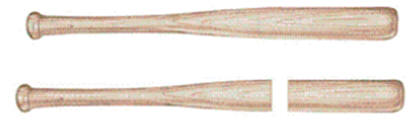After the cut, which piece has the smaller mass?

Solution:

• Reason it out yourself and then watch this video clip.  Was your answer correct?